Statement Questions

Chapter 5 Class 8 Squares and Square Roots
Concept wise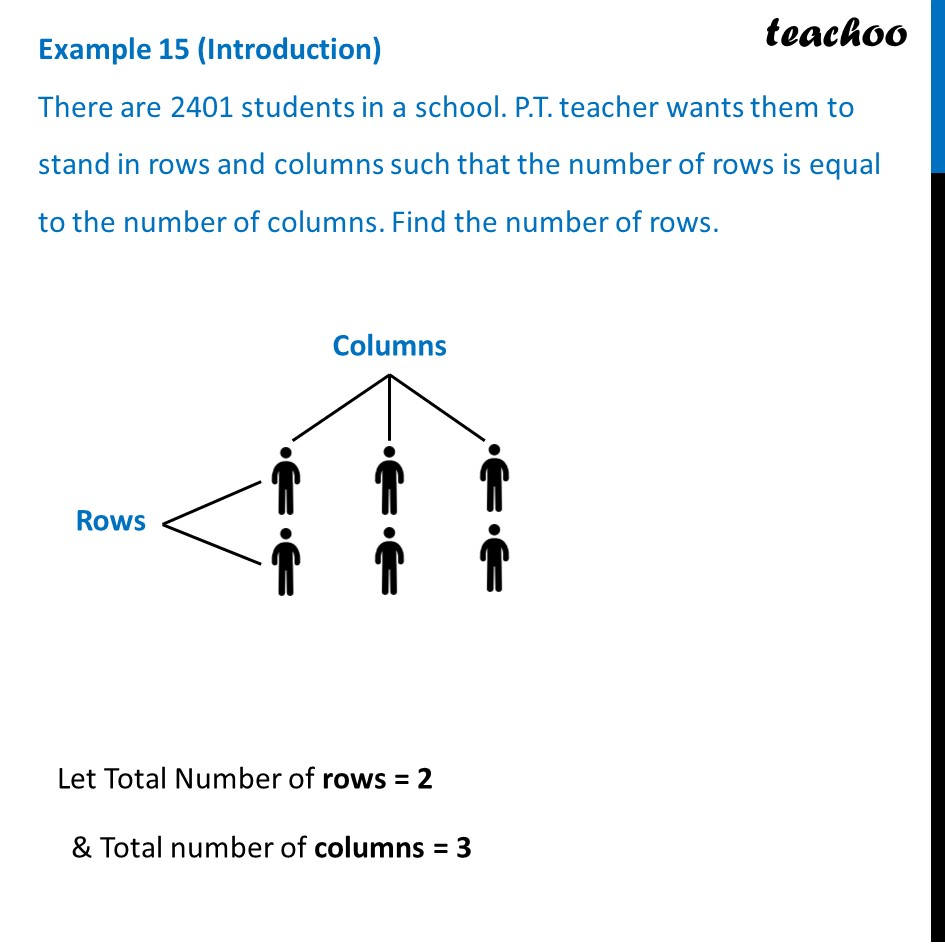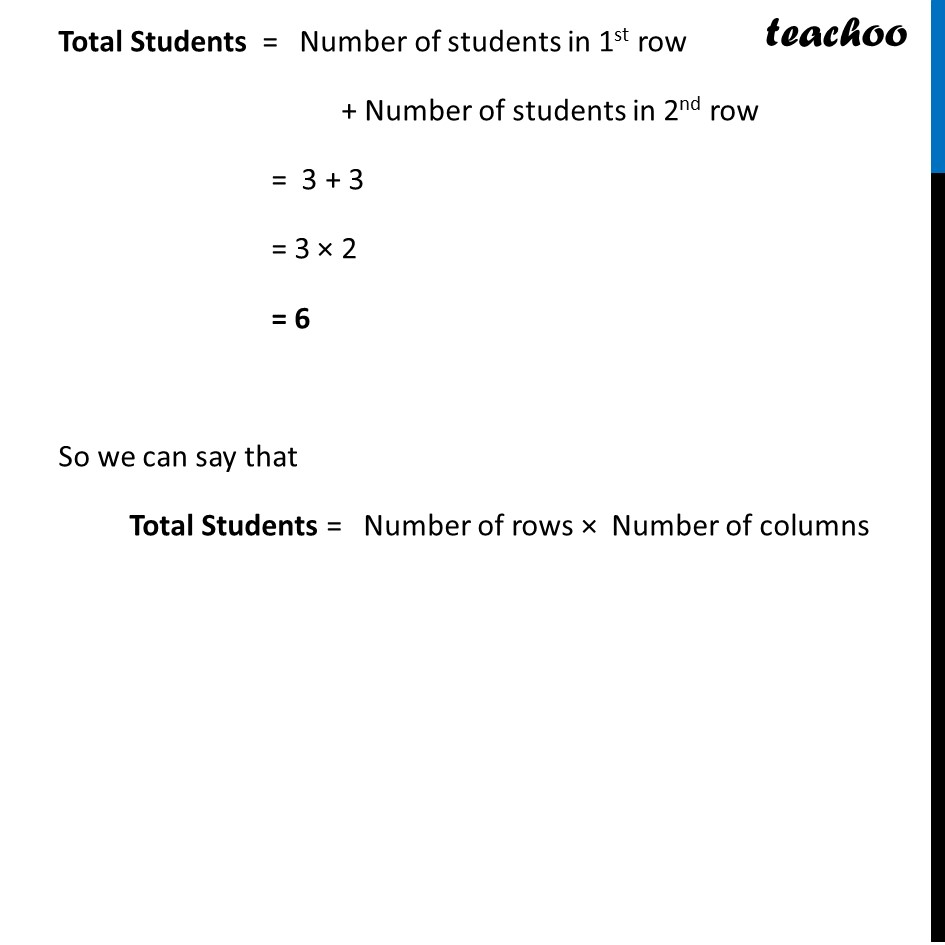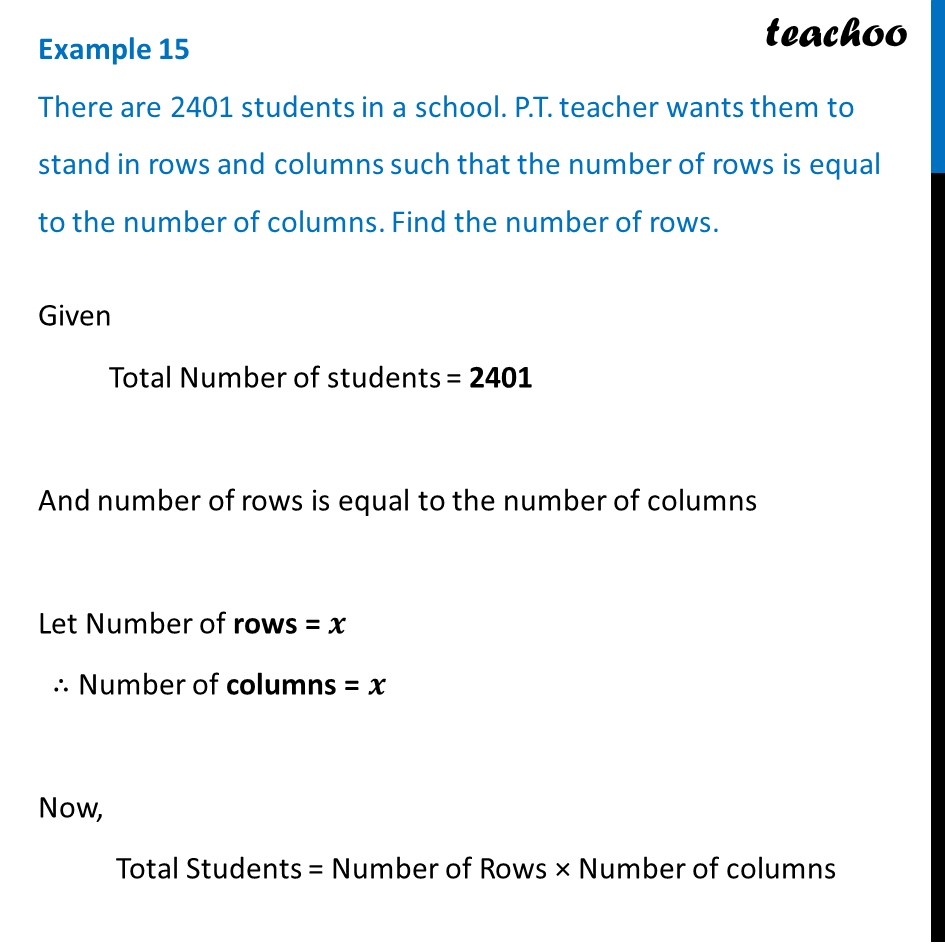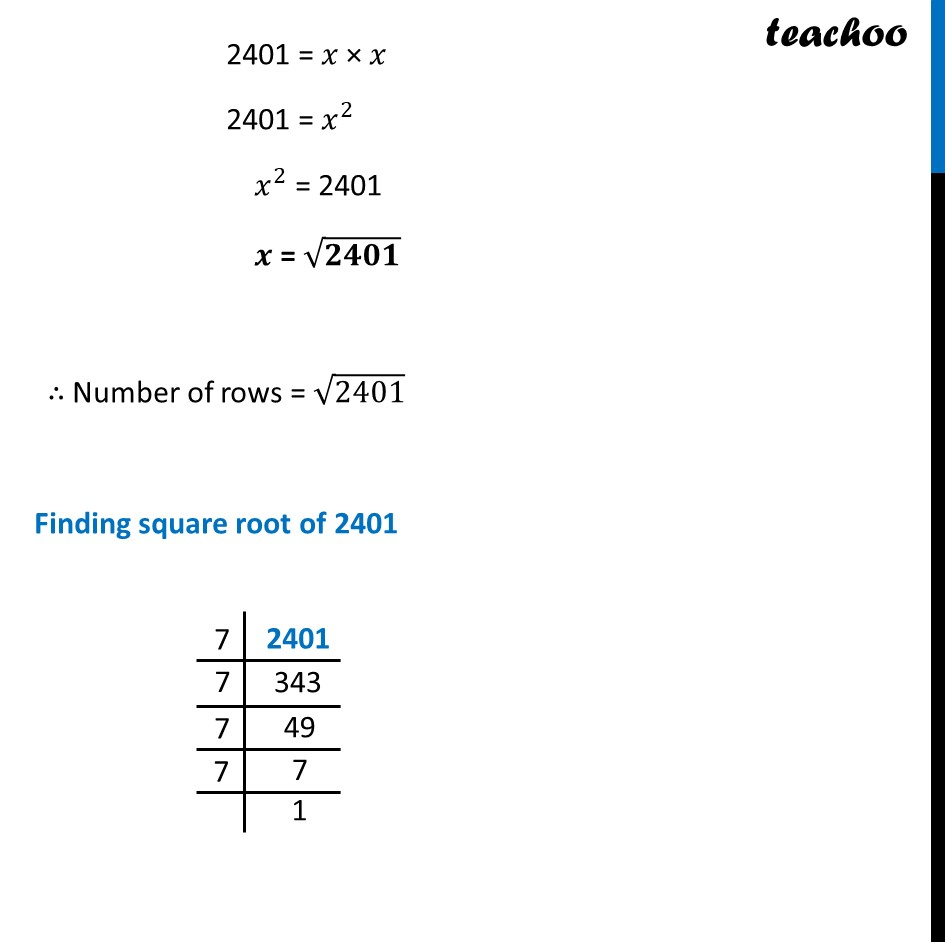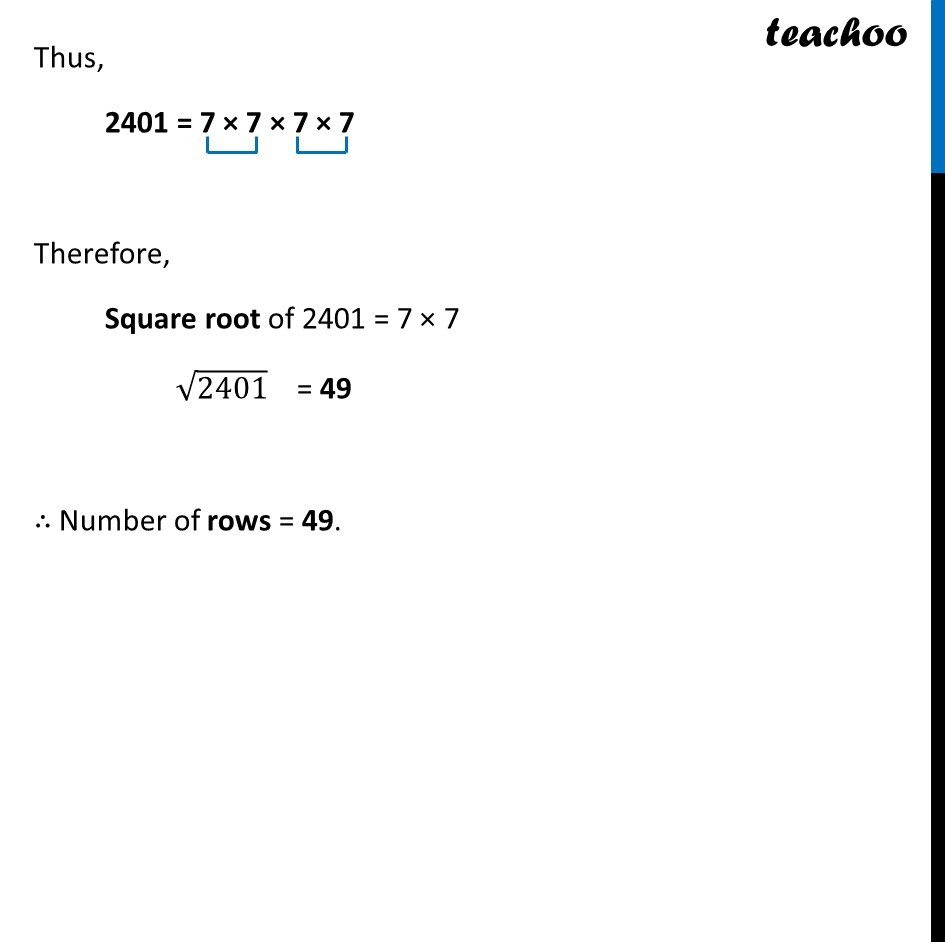Learn in your speed, with individual attention - Teachoo Maths 1-on-1 Class

### Transcript

Example 15 (Introduction) There are 2401 students in a school. P.T. teacher wants them to stand in rows and columns such that the number of rows is equal to the number of columns. Find the number of rows. Columns Rows Let Total Number of rows = 2 & Total number of columns = 3 Total Students = Number of students in 1st row + Number of students in 2nd row = 3 + 3 = 3 × 2 = 6 So we can say that Total Students = Number of rows × Number of columns Example 15 There are 2401 students in a school. P.T. teacher wants them to stand in rows and columns such that the number of rows is equal to the number of columns. Find the number of rows. Given Total Number of students = 2401 And number of rows is equal to the number of columns Let Number of rows = 𝒙 ∴ Number of columns = 𝒙 Now, Total Students = Number of Rows × Number of columns 2401 = 𝑥 × 𝑥 2401 = 𝑥^2 𝑥^2 = 2401 𝒙 = √𝟐𝟒𝟎𝟏 ∴ Number of rows = √2401 Finding square root of 2401 Thus, 2401 = 7 × 7 × 7 × 7 Therefore, Square root of 2401 = 7 × 7 √2401 = 49 ∴ Number of rows = 49.